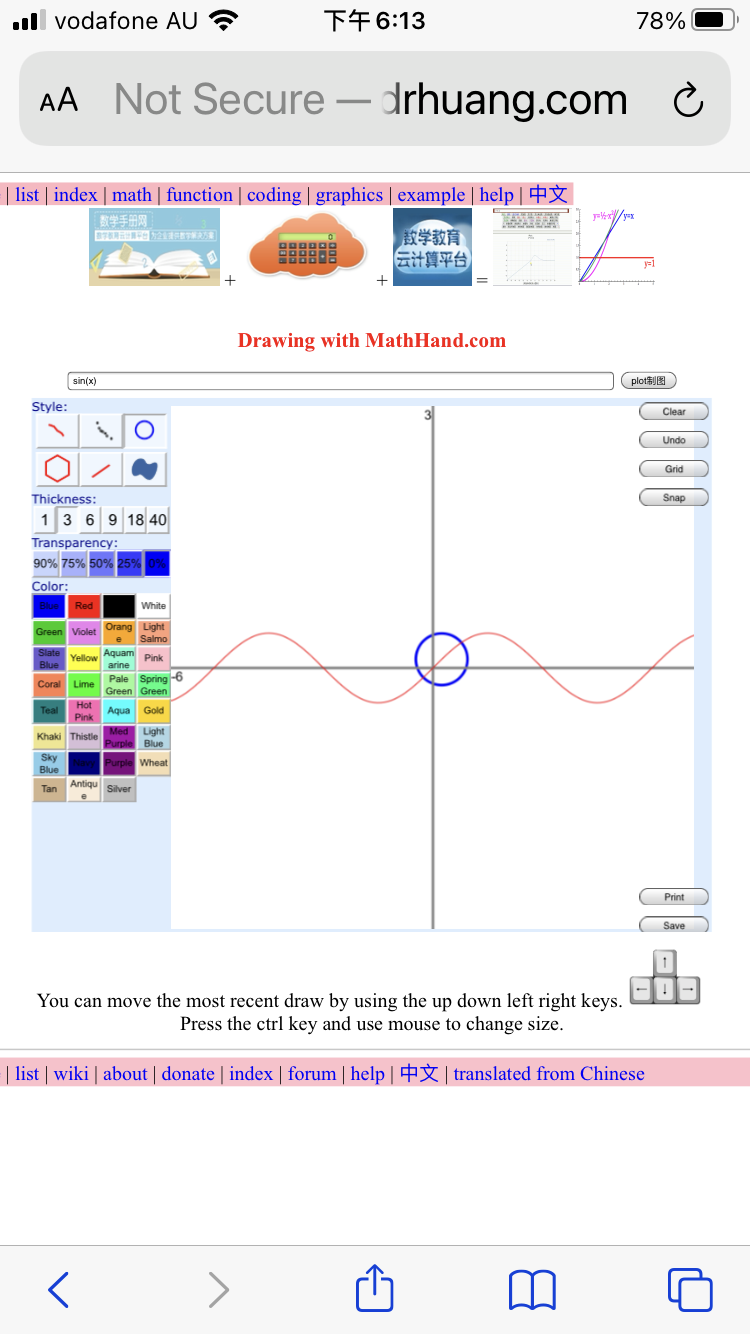﻿ ﻿ Fractional Calculus Computer Algebra System, Electrochemistry Software, chat GPT+++=# 黄博士网 DrHuang.com

 Solar Regional - world - Asia - China Factbook graphics version - text version - flags

## Science

book - Sci-Hub - chat GPT

# Math

• mathHAND.com (former SymbMath) : the Math Handbook Calculator has the function of machine learning. It is unique in the world to solve the function of any order (such as 0.5 order) differential equations. Enter mathematical formulas on the Mathematics Handbook website, click continuously to calculate calculus, solve equations, give analytical solutions and numerical solutions and diagrams, interactively zoom in the drawing, and zoom in with the mouse wheel. You can use it on your mobile phone to learn computing and development anytime, anywhere.
• mathHandbook.com : math handbook
• example - graphics

# Chemistry

• Electrochemistry.net : Electrochemistry net
• ElectrochemistrySoftware.com: electrochemistry software
• Electrochemist.com (former Polar and Polarograph) : virtual electrochemist who can analyze and simulate electrochemical experiments. It simulates voltammetry and chronoamperometry on virtually any mechanism by virtually any waveform techniques. It plots and analyses any x-y data for peak location, peak height, peak width, semi-derivative, derivative, integral, semi-integral, convolution, deconvolution, curve fitting, and separating overlapped peaks and background.

# Medicine

## Rent, sale, buy or auction

### Web statistics for all of drhuang.com

 daily page views ≈ 34000 hits/d (hits per day) (based on Alexa estimates, as of 30/11/2022) daily visitors ≈ 34000 visits/d (visits per day) (based on Alexa estimates, as of 30/11/2022) site rank ≈ 111263th domain online 10/08/2000 (≈22 years ago) traffic fraction 1 in 50 millions of all web traffic
(based on Alexa estimates, as of 30/11/2022, and assuming 4.209 billion global internet users)